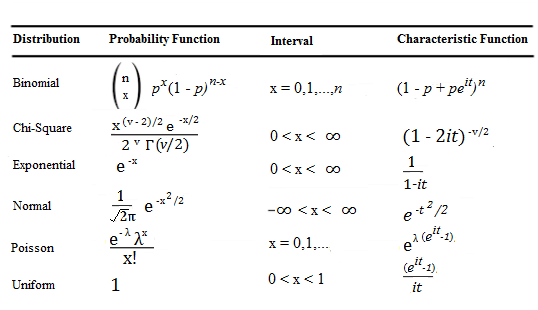# Characteristic Function / Fourier Transform: Simple Definition

Note: the term characteristic function is sometimes called an indicator function. This can cause some confusion, as an indicator function is also a specific function used in set theory. In addition, if you’re looking for the indicator functions used in probability and set theory (that take on values of 1 or 0), see: What is an indicator function? Otherwise, read on.

Contents:

## What is a Characteristic Function?

A characteristic function completely defines a probability distribution. Completely defining a probability distribution involves defining a complex function (a mix of real numbers and imaginary numbers).

The characteristic function φ(X) of a random variable X is:

φ(X) = E(eitX) = E(cos(tX)) + iE(sin(tX))

Where:

## Finding Characteristic Functions

Some of the more common functions (e.g. the normal distribution and binomial distribution) have already been defined (adapted from Lee & Lee, 2010):Finding others can be more challenging, but some rules have been formulated (much in the same way a set of rules for finding derivatives of functions have been found in calculus). For example, if a function is the sum of two independent random variables X and Y, then φ(X,Y) = X + Y (Battin, 1999).

## Fourier Transform

Outside of probability (e.g. in quantum mechanics or signal processing), a characteristic function is called the Fourier transform. The Fourier transform in this context is defined as as “a function derived from a given function and representing it by a series of sinusoidal functions.” In other words, it’s a recipe (made up from sinusoidal functions) for a specific function of interest.

## Characteristic Function vs. MGF

A characteristic function is almost the same as a moment generating function (MGF), and in fact, they use the same symbol φ —which can be confusing. Furthermore, the difference is that the “t” in the MGF definition E(etx) is replaced by “it”. In other words, the imaginary number is not present in the definition of an MGF. Therefore, the characteristic function has the advantage that it always exists—even when there is no MGF.

References:
Richard H. Battin. (1999). An Introduction to the Mathematics and Methods of Astrodynamics. AIAA.
Cheng-Few Lee, John Lee. (2010). Handbook of Quantitative Finance and Risk Management. Springer Science & Business Media.

CITE THIS AS:
Stephanie Glen. "Characteristic Function / Fourier Transform: Simple Definition" From StatisticsHowTo.com: Elementary Statistics for the rest of us! https://www.statisticshowto.com/characteristic-function-fourier-transform/